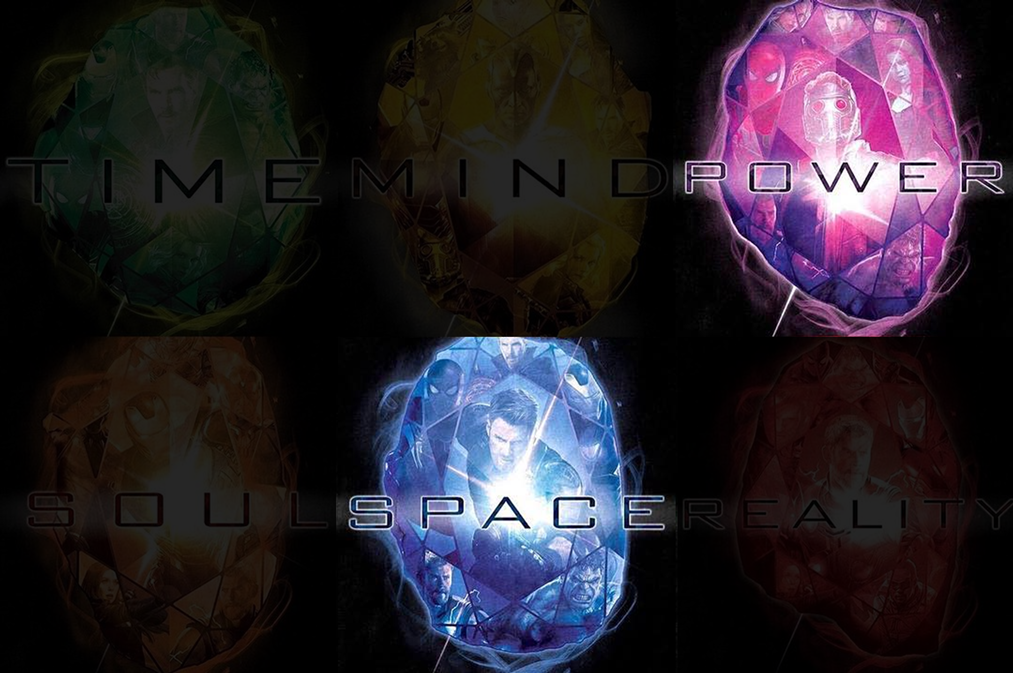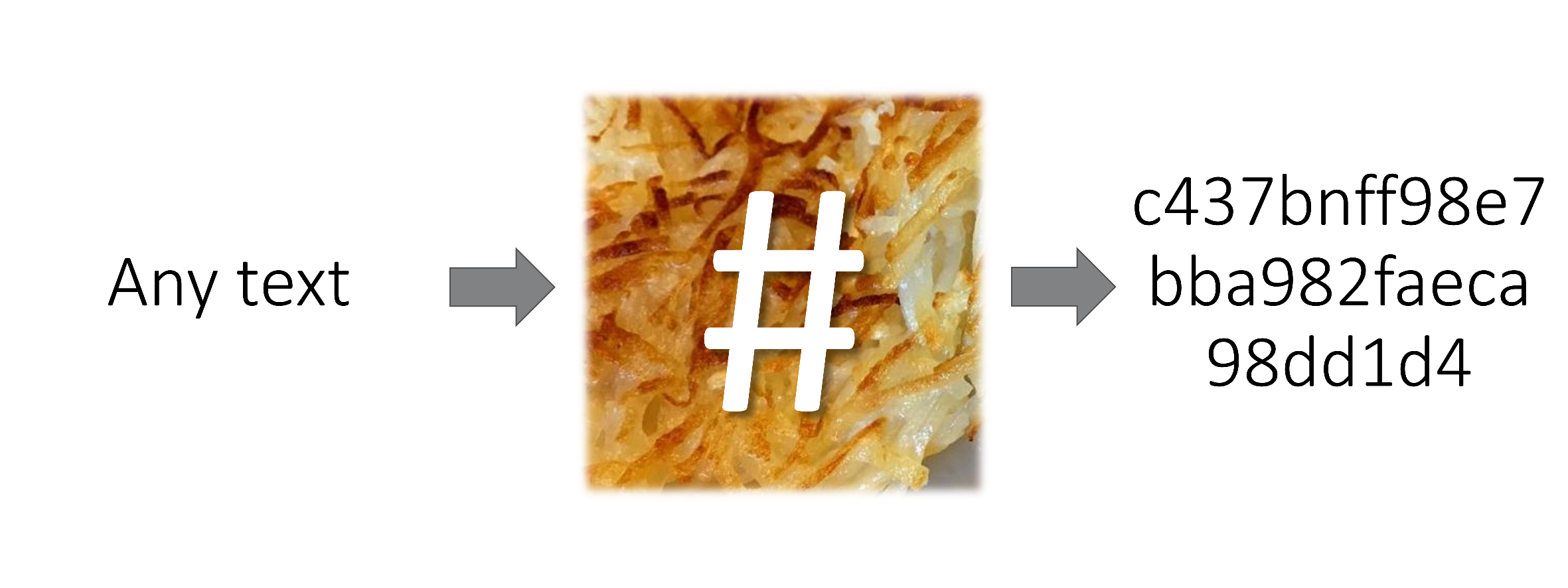# Non-cryptographic hashing functions: The Infinity Stones of Computer Science – Part 1

The new number 2: “You are number six”

Number six: “I’m not a number! I am a free man!”

The new number 2: “Hahahahaha”

The quote above (The Prisoner 1960s TV series) highlights an extreme form of hashing, I’ll grant you that. However, that is after all, a form of hashing – turning something (or someone in this case) into a number.

The new number 2 must have been a computer scientist because he is laughing his head off at number six’s accusing objection. Number 2 was clearly looking at a much bigger picture!

How powerful can such simple concept possibly be? In this three-parts article I will argue that hashing functions are Computer Science’s own Infinity Stones!## The Power and Space Stones

### The scourge

And all the computer scientists rose, shaking their fists at the air shouting in one voice: “Curse ye, dimensionality!

Who hasn’t seen that happening at one time or another, right? The simplest of algorithms can become intractable as the size of the input grows larger.

Intractable usually means one of two things:
1 – “The sun will implode before the algorithm returns” (a matter of time, something we will deal with a later time…)
2 – “We need a bigger boat” (a matter of space)

Consider the case of counting the number of unique items in a list. Very simple to accomplish:
– create a new empty list,
– go through the original list one item at the time,
– only add the next item from the original list to the new list if it is not already there, and
– count the items in the new list.

In the worst case scenario the new list may end up as big as the original and if the latter is huge to begin with, bye bye memory!

Who cares about counting unique items in a list anyway? Only every database developer on the planet and you, the moment you utter “SELECT  count(DISTINCT X) FROM Y WHERE Z“.

## Counting random numbers… somewhat

Suppose we have M balls in a jar, each ball distinguishable by its colour and we want to find out how many different colours we have in total by drawing balls at random from the jar.

We could setup N bins and put a 1 in bin j if we have drawn at least one ball of colour j.

If we sum all the 1s across all bins we should have the number of unique colours observed so far.

Let’s now replace balls with numbers in their binary representation for convenience, that is as strings of 1s and 0s of length L.

Furthermore let’s pretend that a number’s “colour” corresponds to how many 0s are found at the beginning of this number. For example, a number starting with 0001…. (i.e. three 0s) is of colour 3, 0000001… is of colour 6 and 1……. is of colour 0.

We get our bins ready once again and start drawing numbers at random setting bin j equal to 1 every time we draw a number of “colour” j.

Imagine that after a while we observe a number n of unique colours. We can tell how many times we expect bin j to have been visited. Steady on, now.

## Probability interlude

By construction, the probability of drawing a number of colour, say, 3, is the same as the probability of a number starting with 0001…

This probability is easy to estimate: it’s the probability of flipping a coin and getting a 0 (tail) followed by another 0, then another 0 and then a 1 (head).

With a fair coin the probability of flipping a 0 is 1/2, the same as the probability of flipping a one. Therefore, the probability of drawing a number that starts with 0001.. is 1/2 * 1/2 * 1/2 * 1/2 or in more mathematical notation 2−(j−1).

Take a deep breath.

So how many times do we expect bin 3 to have been visited given we have observed n unique colours?

It’s the probability that the number was of colour 3 and that it was the first unique colour we observed or that the number was of colour 3 but it was the second unique colour we observed or that the number was of colour 3 but it was the third unique….you see where I am going with this.

Turn “or”s into “+”s and the expected number of visits to bin 3 is n*2-(3-1). And for j = 6? n*2-(6-1) and in general n*2-(j-1).

Notice that the lower the value of j the more probable it is that we visited bin j, conversely, the higher the value of j the more improbable the visit.

We find a sweet spot at around j = log2 n. This sweet spot value of j is roughly the index of the first bucket, in order, that had a 0 in it (i.e. not have been visited). In other words, we have most likely observed n = 2j unique colours.

This is awesome! it means we can estimate how many unique numbers there are in a set drawn at random by tracking which of the observed numbers has the most leading zeros, call this number of 0s j and the estimate is simply 2j.

If only we could turn other things into numbers!## Hyper what ?!

Here we finally discover how hashing functions gift us the abilities of the Power Stone and the Space Stone.

Hashing functions let us turn anything we want to count (words, records, documents) into numbers which, in conjunction with the above observations become the basis for the impressively named HyperLogLog algorithm.

Hashing records in a database table for example, effectively turn the table into a set of random numbers and HyperLogLog can estimate the number of unique records (cardinality) by looking at these numbers’ leading 0s in the hashes.

Space is no longer an issue (Space Stone) as the amount of memory required only depends on the number of bins we need.

It destroys (Power Stone) the space requirements of more conventional solutions by an order of Log of the Log of the cardinality of the multiset under consideration (the inverse of a Power operation) as the name suggests- although for the life of me I still don’t get what’s Hyper about it.

Wikipedia reminds us that it is able to estimate a cardinality of up to 109 using only 1.5 KB of memory with an accuracy of 2%.

HyperLogLog is more sophisticated than the probabilistic counting we explored earlier in how it constructs the bins (registers) and how it averages across them.